# Assignment

1. your previous knowledge in some of Machine Learning methods we use and their code implementations
3. your skills on understanding and using methods that you may not be familiar with.

## Sensors

In raw/ there are csv files with time series data of inertial sensors installed in a car:

1. accelerometer (x, y, z) at 100Hz and 200Hz sampling rate
2. gyroscope (roll, pitch, yaw) at 200Hz sampling rate

Which we load with this script.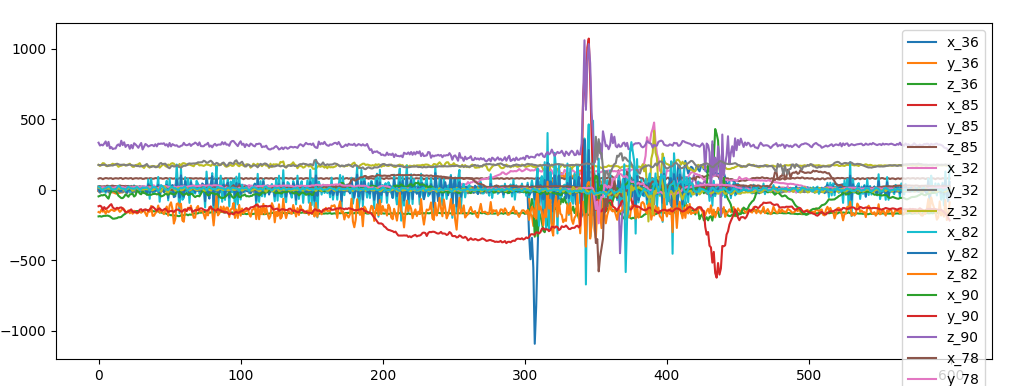superposition of sensor data for accellerometer

Without further information (timestamp and position of the sensor) we can't assume that sensors are alligned in space and synced.

Still sensors might be aligned: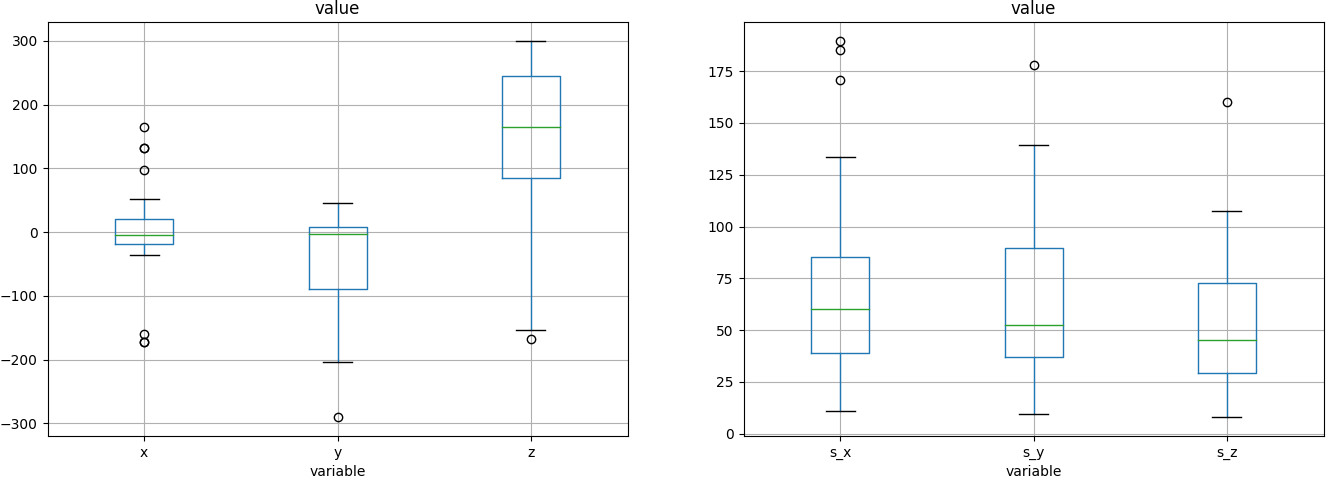similar acceleration between sensors

We can suppose that y adds gravity, z is the direction of motion and x is the lateral displacement and we can reconstruct the equation of motion and see that the car is accellerating (probably we should remove the constant gravity).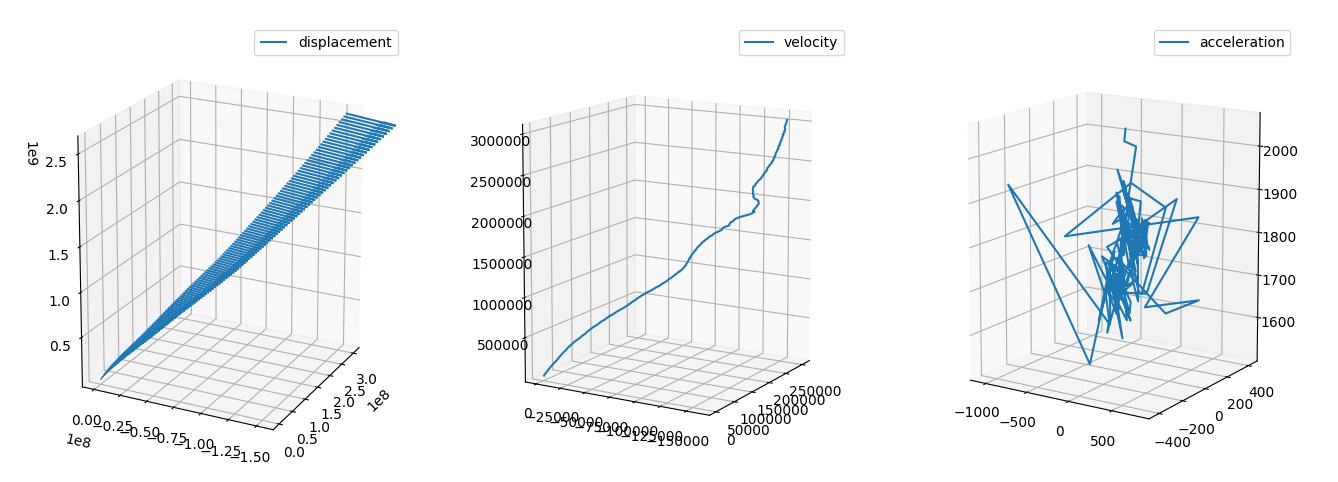equation of motion

To capture the most essential feture of a time series we first smooth and calculate the derivatives of the time series.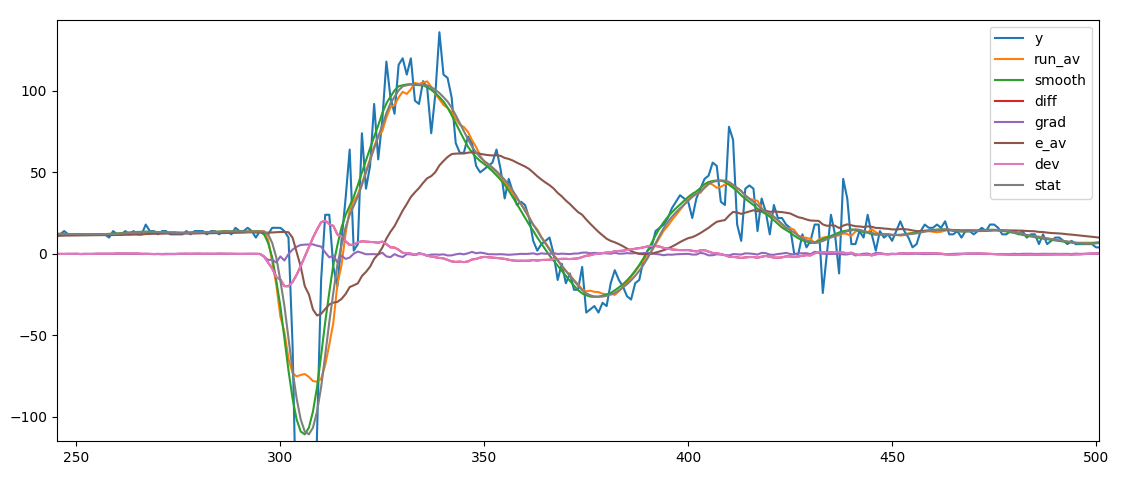smoothing of the series

The single series are calculated as follow:

serD = pd.DataFrame({'y':y})
serD.loc[:,"run_av"] = serRunAv(y,steps=period)
serD.loc[:,"smooth"] = serSmooth(y,width=3,steps=period)
serD['diff'] = serD['smooth'] - serD['smooth'].shift()
serD['e_av'] = serD['y'].ewm(halflife=period).mean()
serD['stat'] = serD['run_av'] - serD['diff']

Kaiser window smoothing delivers more stable results but running average is computationally more efficient. Derivaties are as well computationally really efficient (float difference).

After smoothing we can sample the signal (i.e. take every 3rd)

### Unsupervised classification of events

We analyze at first every single sensor independently and we classify the events

Since we have smoothed the signal we keep every third data point to reduce the size of the learning set. We create a series of segments chopping the signal into sequential windows of 16 data points.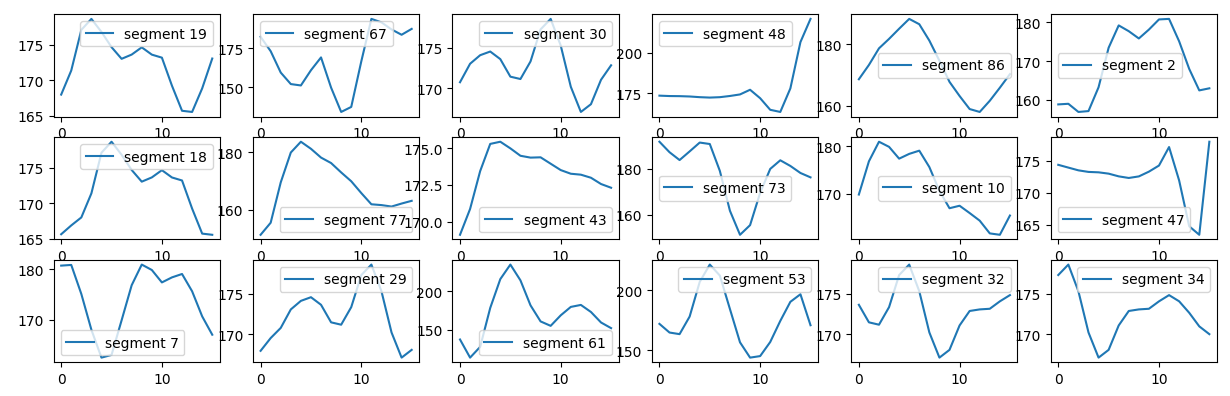training set for unsupervised shape classification

We use sklearn kmeans cluster to clusterize the shape.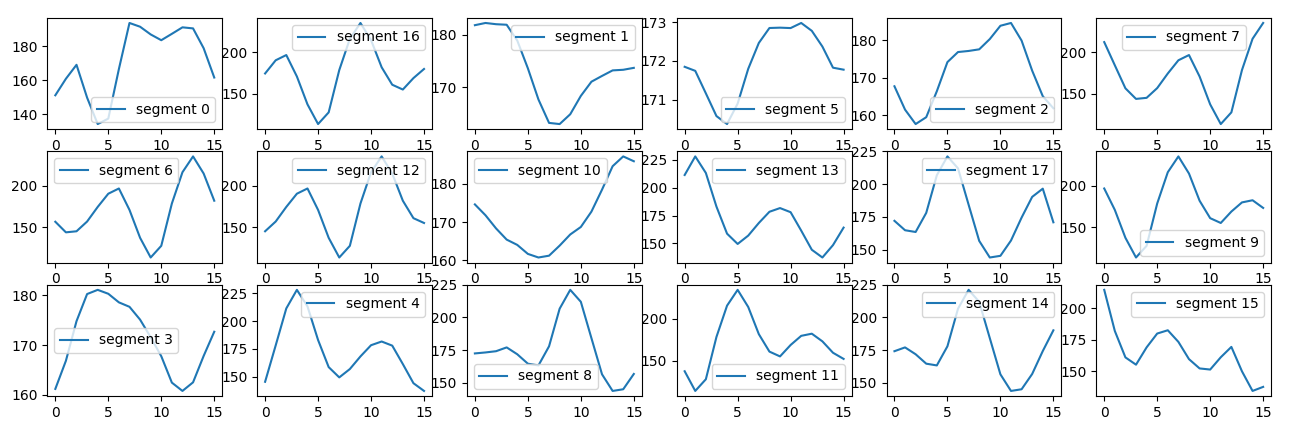set of shapes from the k-means fit

Each cluster should have a physical meaning and can be ad hoc adjusted

Each time series is now represented by a string of k-clusters:

• sensor_id_36_ACC_100Hz : 'sdtssssstfswfw'

### Represent inertial sensor data with reduced information and as accurate as possible.

We see in some cases an high correlation between clusters which mean we can reduce the size of k.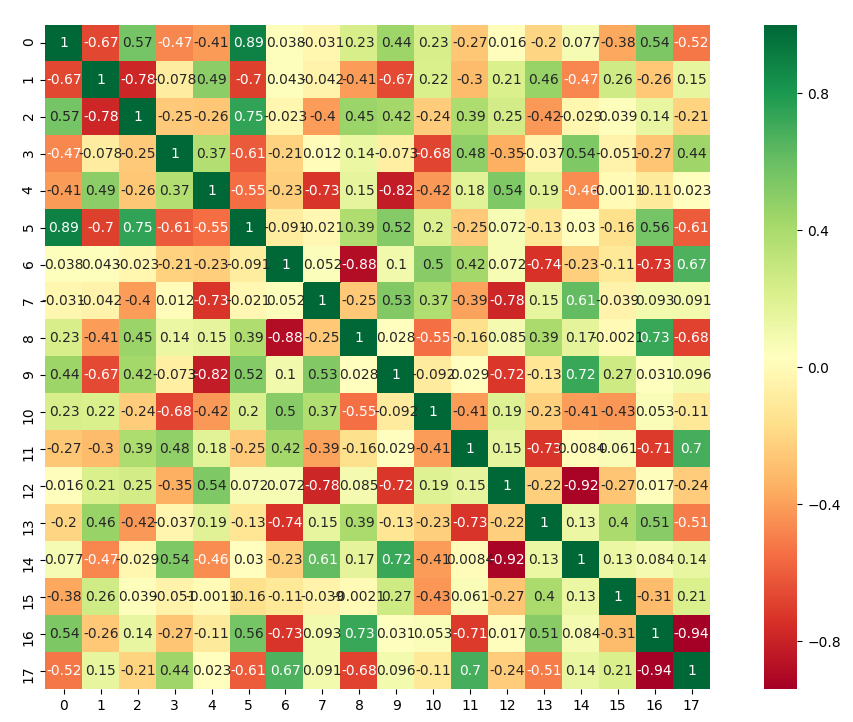high correlation or anticorrelation between some clusters

We can than evaluate in which cluster each segment belong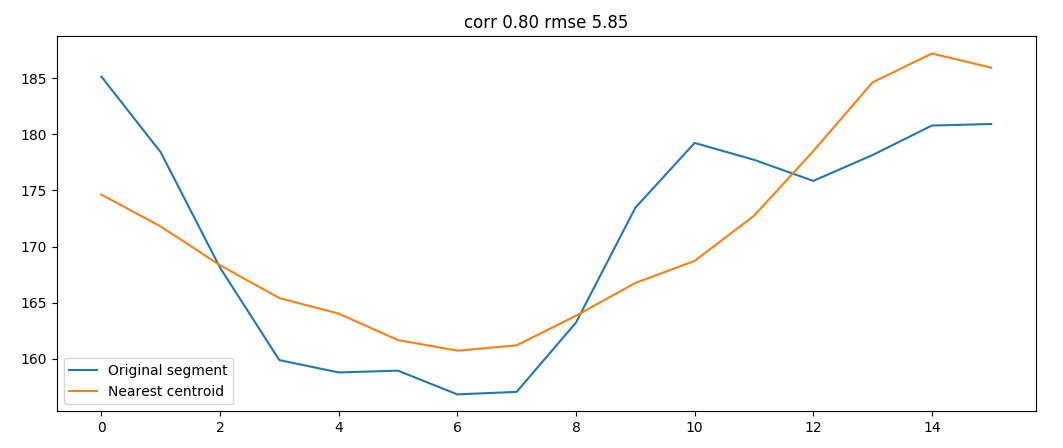original segment and correspondent cluster

We can than reduce a window into a single class saving a lot of information

### Most important signal categories

The power spectrum can with few data points convey many important information

• Periodicity
• Strength
• Noise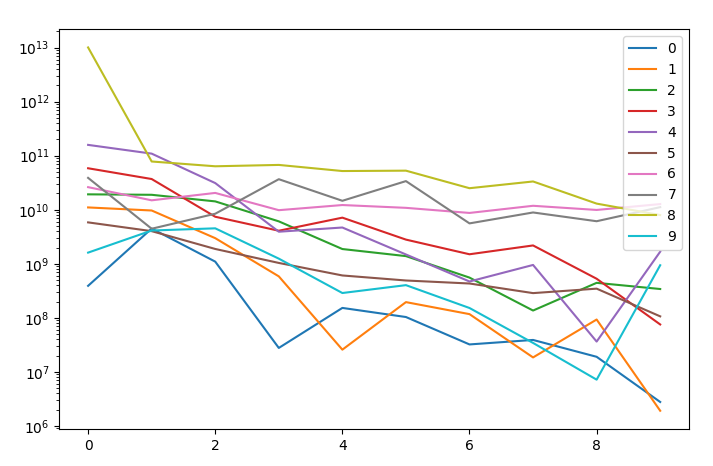comparison of the power spectrum of few signals

There is a strong correlation between many sensors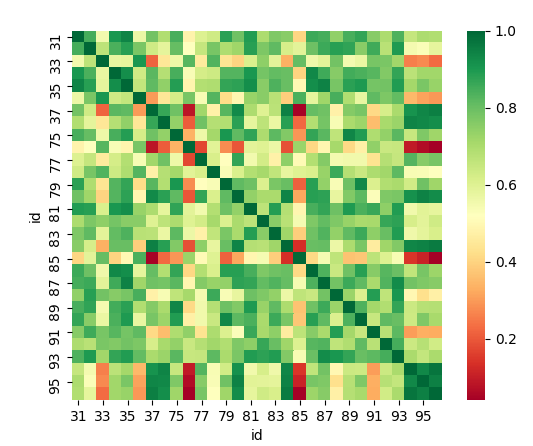many sensors are higly correlated

If we consider each direction taken separately we obtain a lower correlation which might reveal that some sensors might be flipped.correlation from power spectrum, sum of directions

Power spectrum helps against redundancy

Power spectrum is an heavy computation and can be done at best in the communication design.

A similar analysis might be done with other statistical features of a sensor:

• mean
• standard deviation
• percentiles

Further statistical properties should be added since the correlation matrix doesn't show useful interpreting results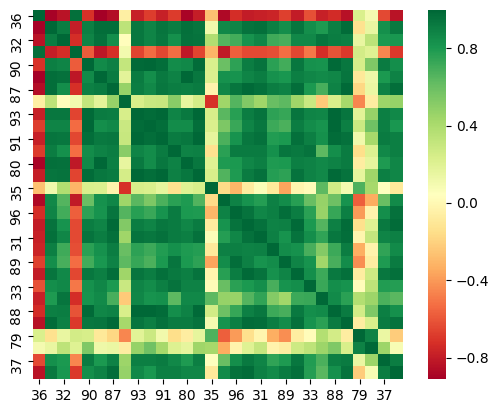correlation of statistical properties

### optimizing the network

Once we establish a correlation between sensors we can build a graph and understand how to simplify the information across the system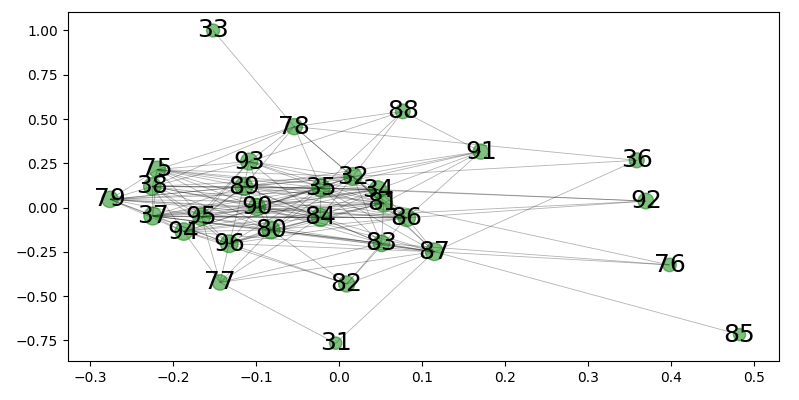network from directional spectrum

Different analysis lead to different results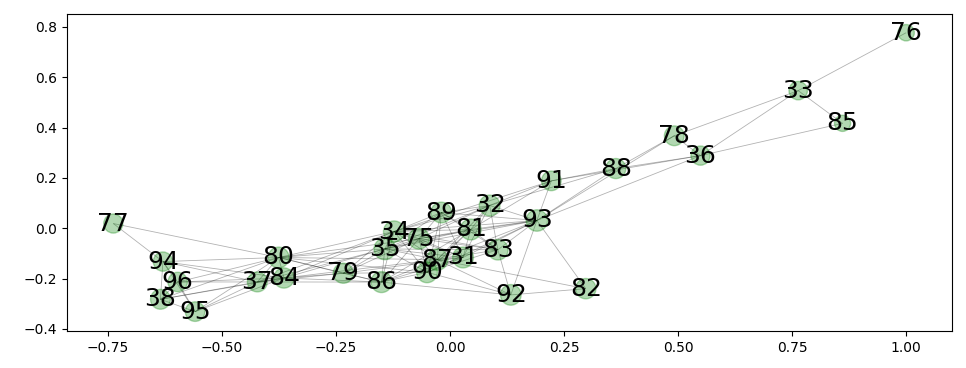network from radial spectrum

## Spikes classification

### Event classification

Depending on the functionality of the sensor we can decide to report only spikes using derivatives and callback functions.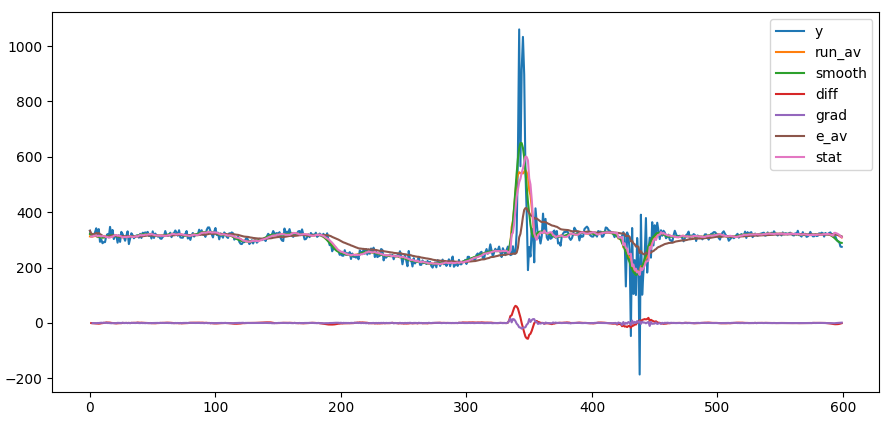the derivative of the accelleration as spike

Derivaties should trigger a window and the event classification letting the sensor just send a single category + timestamp.

### spike definition

For this particular exercise we want to derive from the power spectrum the presence of a spike.

We first build a spike detector

y = sen[l].values
serD = s_l.serDecompose(y,period=16)
serT = s_l.serDecompose(serD['diff'].abs(),period=16)
spik = serT['smooth'].values > np.mean(serT['smooth'])*3.

and we than create the training set

    feat = psPx.T.sort_values('id')
spik = spL.sort_values('id')['x'] > 0
X = feat.values
y = spik.values * 1

We than use the two methods mentioned in the assignement (support vector classifier - sklearn, neural network - keras) and compare the performances:

• svc : accuracy 100%
• neu1 : accuracy ~ 90%
• neu2 : accuracy ~ 90%

Neuronal network with the given topology are much slower and less performant than support vector machine. I some cases the network get trapped in a local minimum and does not find the solution.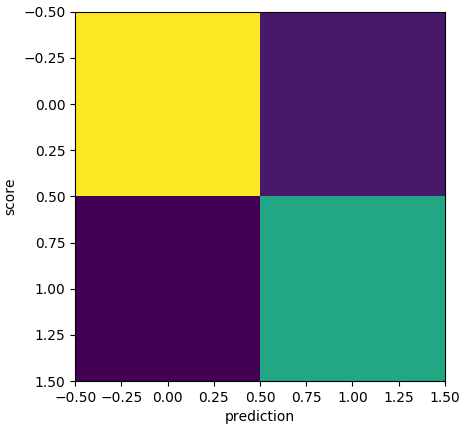confusion matrix for the neural network when trapped in a local minimum

### Learning capability

We want to understand which is the learning capability of a cluster of sensors to create local processing nodes which summarize the information.

Since convolutional neural networks are really performant we transform our time series into images usinig a characteristic period (from power spectrum)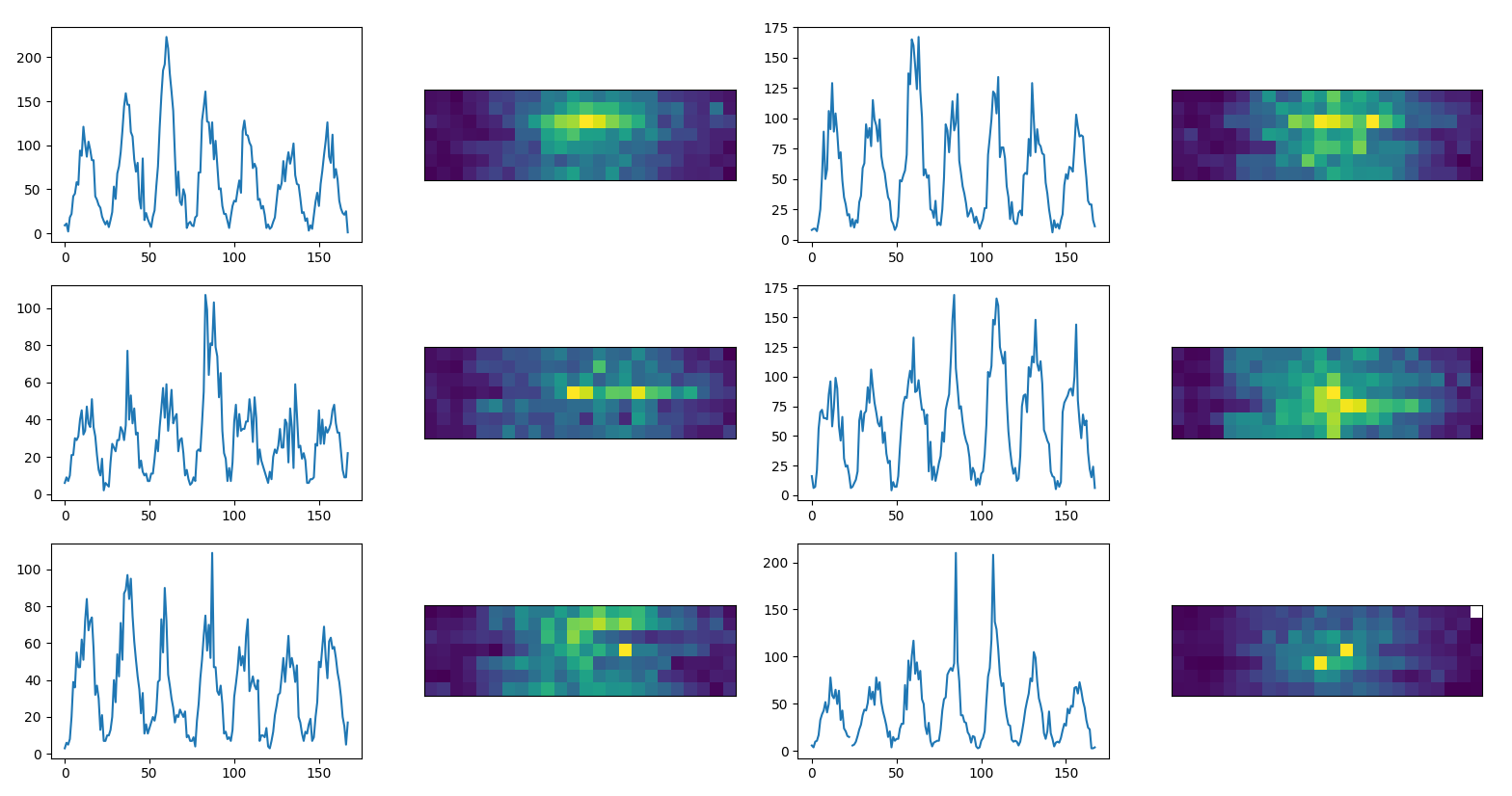time series as images (example data)

and we write an autoencoder to predict each image and calculate performances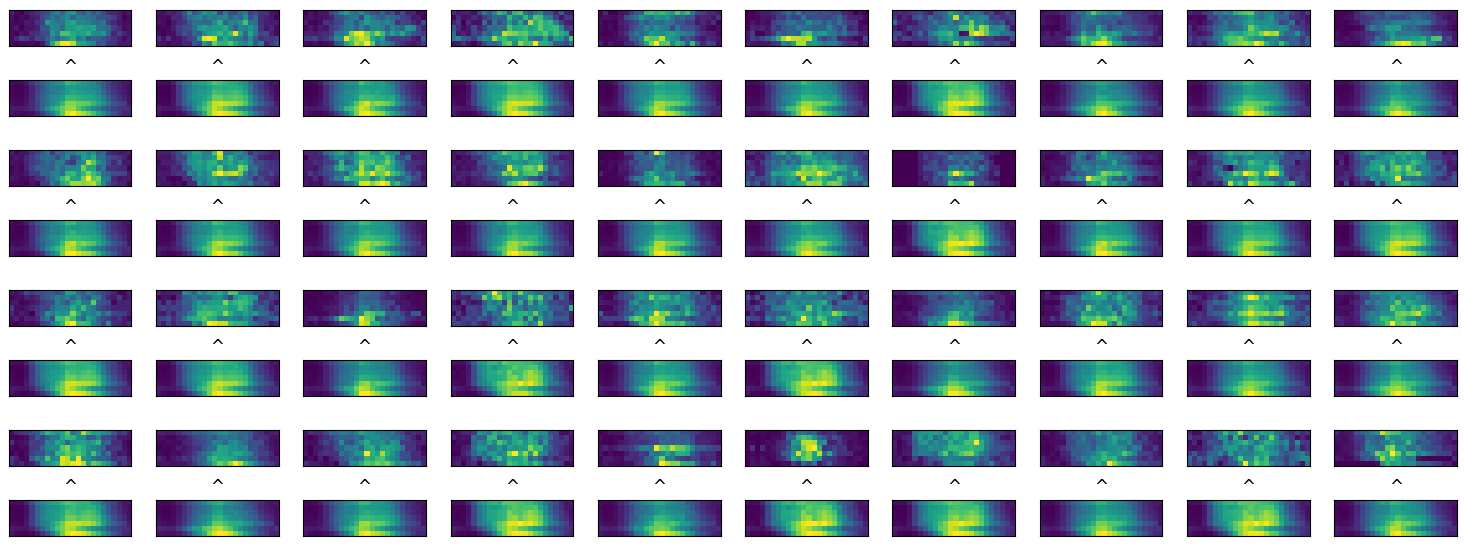comparison between original time series and their predictions (example data)

We take a set of sensors into a cluster node and we predict one time series with the help of the others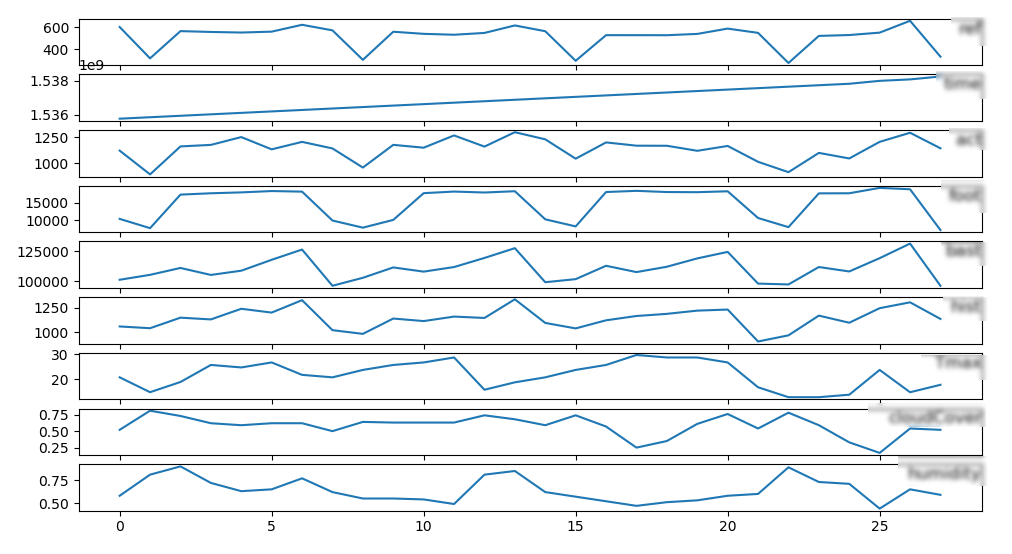series of data for learning (example data)

We than perform a long short term memory learning with keras and study the influence of each sensor into the predictionperformances on predictions after feature knockout (example data)

## Further classification

There are different algorithms that can be tested for classify the signals. Among the most interesting we point to:

## TODO

There is a list of further analysis to do to investigate further information in the system:

• Average 200Hz sensors to compare them with 100Hz
• Compare the correlation results against mutual information scoring with sklearn
• unsupervised pattern learning after independent component analysis
• causality between sensors: is there a short time delay between sensor events?

## Summary

1. Are there any categories in which you can classify the accelerometer/gyroscope datasets?
• statistical properties: mean, std, percentiles
• power spectrum: first modes
• presence of spikes
• predictability
• categorical unsupervised classification of time windows
1. Which are the suitable feature(s) used for this classification?
• power spectrum
• kmeans
1. Which technique would you use to classify the signals and why?
• spikes: support vector classifier, good performances, fast calculation
• segment cluster: KMeans
1. Are there any intra-signal correlations? If so, do they help in the classification task?
• reduce the network dimension
• clusterize sensors

Part 2

## Dictionary learning

sklearn provides an api and doc for dictionary learning.

Now we have time series expressed as strings and we want to: * Finds a dictionary (a set of atoms) that can best be used to represent data using a sparse code.

### Summary

Given goal (b) in the context of a sparse coding problem:

1. Is Dictionary Learning a good approach and why?
2. Which would be the indicators that a Dictionary Learning approach works well?
3. Do the answers of Part 1 help to reach the Goals?
4. Could you draft how to speedup or reduce the computational complexity of the sparse coding problem?

You may use sklearn Dictionary Learning for the sparse coding part. Which other tools/methods would you suggest?

## Questions

1. Suppose you have trained a support vector machine classifier which takes as an input feature vectors of length N and outputs a boolean variable. Now, in order to perform lassification on new data samples, you want to store trained parameters of your model using as little memory as possible. How much memory would you need? What does it depend on? Consider cases of linear and non-linear kernels.

A Kernel function K in support vector machine acts like an inner product in a transformed space 1 2 3 4 5

K(x, x′) = <ϕ(x),ϕ(x′)>

The number of parameters depend by the augmented dimensionality induced by the kernel. In case of a linear kernel ϕ can be expressed with a N number of parameters, for non linear kernels it depends whether we use a polynomial, gaussian, or gaussian radial kernels 6.

In general the kernel acts in a M-dimensional space operating on the N features, where M > N, to find a linear classification in the M dimensional space.

From sklearn we can extract the attributed of the kernel

• support_vectors_ : array-like, shape = [n_SV, n_features]
• n_support_ : array-like, dtype=int32, shape = [n_class]
• coef_ : Weights assigned to the features (coefficients in the primal problem). This is only available in the case of a linear kernel.
1. Suppose you have a neural network for binary classification. The input layer contains 20 nodes, and there are two hidden layers of sizes 10 and 5. All layers are fully connected. What are the shapes of the weights of this network? How many parameters are in the network in total? Which activation functions would you use 1) in the intermediate layers 2) in the final layer

We create a fully connected network (no drop out) with 3 layers:

from keras.models import Sequential
from keras.layers import Dense
model = Sequential()
model.add(Dense(1, activation='sigmoid'))
• We pick the rectifier activation function for the first two layers for better performances, low saturation but can easily disconnect a neuron 1 2
• We use a sigmoid on the output layer for an output probability range (normalized) and a binary classification
• good for binary_crossentropy loss function 2

We can then complile the model and test different optimizer and loss functions:

model.compile(loss='binary_crossentropy', optimizer='adam', metrics=['accuracy'])

In our specific case we try as well this other combination

    from keras.utils import to_categorical
y_binary = to_categorical(y)
model = Sequential()
model.fit(X, y_binary, epochs=150, batch_size=10)
y_pred = np.array(model.predict(X) > 0.5)[:,1]
print(sum( abs(y*1-y_pred*1) ) )

We see that both models sometimes get trapped in a local minimum and still don't perform as much as support vector machine which in few milli seconds always get to the correct solution.

### Strings

You are given two strings. Write a Python function which would check if one of them can be obtained from the other simply by permutation of characters. The function should return True if this is possible and False if it is not possible. Example: for the pair (“qwerty”, “wqeyrt”) it should return True and for the pair (“aab”, “bba”) it should return False.

import string
def isPermuted(w1,w2):
cL = [c for c in string.ascii_lowercase]
d1, d2 = {}, {}
for c in cL:
d1[c], d2[c] = 0, 0
for w in w1.lower() :
d1[w] = d1[w] + 1
for w in w2.lower() :
d2[w] = d2[w] + 1
return d1 == d2

print(isPermuted("qwerty", "wqeyrt") )
>>> True
print(isPermuted("aab","bba"))
>>> False